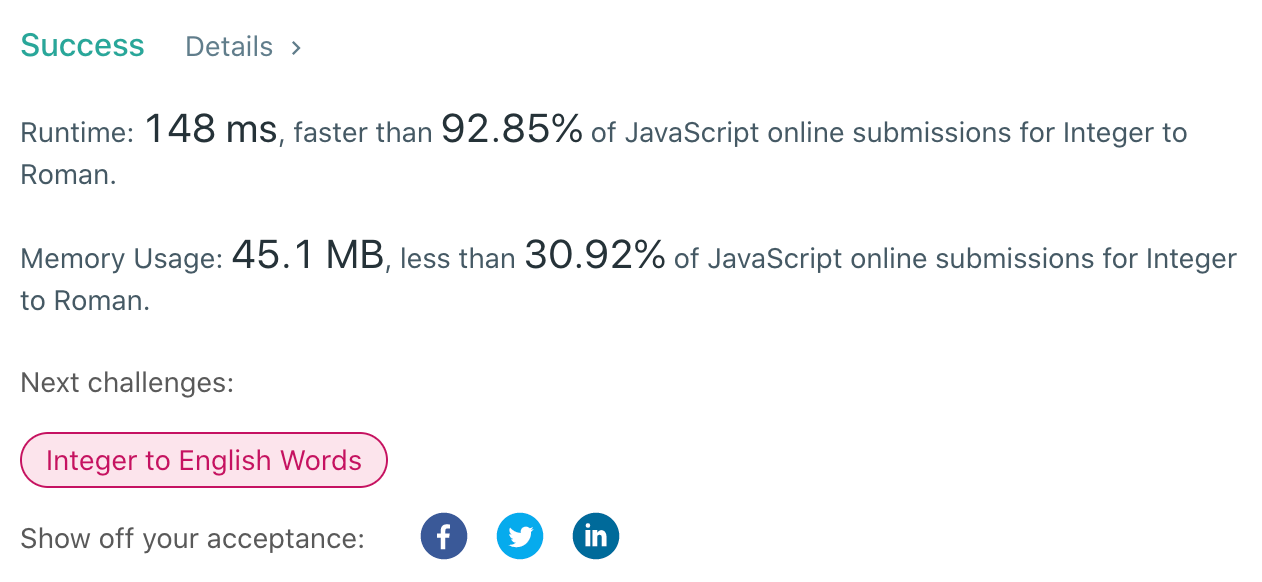# JavaScript Algorithms: Integer to Roman (LeetCode)

### Description

Roman numerals are represented by seven different symbols: `I`, `V`, `X`, `L`, `C`, `D`, and `M`.

``````**Symbol**       **Value**
I             1
V             5
X             10
L             50
C             100
D             500
M             1000
``````

For example, `2` is written as `II` in Roman numeral, just two one's added together. `12` is written as `XII`, which is simply `X + II`. The number `27` is written as `XXVII`, which is `XX + V + II`.

Roman numerals are usually written largest to smallest from left to right. However, the numeral for four is not `IIII`. Instead, the number four is written as `IV`. Because the one is before the five we subtract it making four. The same principle applies to the number nine, which is written as `IX`. There are six instances where subtraction is used:

• `I` can be placed before `V` (5) and `X` (10) to make 4 and 9.

• `X` can be placed before `L` (50) and `C` (100) to make 40 and 90.

• `C` can be placed before `D` (500) and `M` (1000) to make 400 and 900.

Given an integer, convert it to a roman numeral.

### Example 1:

``````Input: num = 3
Output: "III"
``````

### Example 2:

``````Input: num = 4
Output: "IV"
``````

### Example 3:

``````Input: num = 9
Output: "IX"
``````

### Example 4:

``````Input: num = 58
Output: "LVIII"
Explanation: L = 50, V = 5, III = 3.
``````

### Example 5:

``````Input: num = 1994
Output: "MCMXCIV"
Explanation: M = 1000, CM = 900, XC = 90 and IV = 4.
``````

### Constraints:

• `1 <= num <= 3999`

### Solution

First of all, we're going to create a `map` with all possible Roman-Integer pairs.

The algorithm is going through this `map` from biggest to smallest starting from `M` to `I` and keeping track of a `result` variable.

The main algorithm is using the division `num` by `map[key]` and modulus operator. The division tells us how many particular symbols do we need to repeat. And the modulus operator helps us to change the `num`.

The easiest way to understand this solution is to look at an example :) Let's imagine we need to convert `2439`:

In this solution, we can do some improvements:

• We can return the result if the num equals `0`

• We can check whether the division equals `0` or not, if not we don't need to repeat anythingThe time complexity is going to be `O(n)`, there is `n` is the number of Roman numeral characters. And the space complexity is `O(1)`.

Hope it was useful for you!